# Pseudo-Linear Lightwave Systems

Pseudo-linear lightwave systems operate in the regime in which the local dispersion length is much shorter than the nonlinear length in all fiber sections of a dispersion-managed link. This approach is most suitable for systems operating at bit rates of 40 Gb/s or more and employing relatively short optical pulses that spread over multiple bits quickly as they propagate along the link. This spreading reduces the peak power and lowers the impact of SPM on each pulse. There are several ways one can design such systems. In one case, pulses spread throughout the link and are compressed back at the receiver end using a dispersion-compensating device. In another, pulses are spread even before the optical signal is launched into the fiber link using a DCF (precompensation) and they compress slowly within the fiber link, without requiring any post-compensation.

A third possibility is to employ periodic in-line compensation. In this case, the dispersion map is chosen such that each pulse broadens by a large factor in the first section but it is compressed in the following section with opposite dispersion characteristics. An optical amplifier restores the signal power after the second section, and the whole process repeats itself. Often, a small amount of dispersion is left uncompensated in each map period. This residual dispersion per span can be used to control the impact of intrachannel nonlinear effects in combination with the amounts of pre- and post-compensation.

As optical pulses spread considerably outside their assigned bit slot in all pseudo-linear systems, they overlap considerably and interact with each other through the nonlinear term in the NLS equation. It turns out that the spreading of bits belonging to different WDM channels produces an averaging effect that reduces the interchannel nonlinear effects considerably. However, at the same time, an enhanced nonlinear interaction among the bits of the same channel produces new intrachannel nonlinear effects that limit the system performance, if left uncontrolled. Thus, pseudo-linear systems are far from being linear. The important question is whether pulse spreading helps to lower the overall impact of fiber nonlinearity and allows higher launched powers into the fiber link. The answer to this question turned out to be affirmative. In this section we focus on the intrachannel nonlinear effects and study how they affect a pseudo-linear lightwave system.

#### 1. Origin of Intrachannel Nonlinear Effects

All pseudo-linear systems suffer from the nonlinear interaction among the neighboring overlapping pulses. Starting in 1999, such intrachannel nonlinear effects were studied extensively. In a numerical approach, one solves the NLS equationfor a pseudo-random bit stream with the inputwhere tm = mTb, Tb is the duration of the bit slot, M is the total number of bits included in numerical simulations. Here, Um governs the shape of input pulses, and Um = 0 if the mth pulse represents a 0 bit.

Even though numerical simulations are essential for a realistic system design, considerable physical insight can be gained with a semianalytic approach that focuses on three neighboring pulses. If we write the total field as U = U1 + U2 + U3 in the NLS equation above, it reduces to the following set of three coupled NLS equationsThese three coupled equations show explicitly the origin of intrachannel nonlinear effects. The first nonlinear term corresponds to SPM. The next two terms result from XPM induced by the other two pulses. Since these terms represent XPM interaction between pulses belonging to the same channel, this phenomenon is referred to as intrachannel XPM. The last term is four-wave mixing (FWM)-like and is responsible for intrachannel FWM. Although it may seem odd that FWM can occur among pulses of the same channel, one should remember that the spectrum of each pulse has modulation side bands located on both sides of the carrier frequency. If different sidebands of two or more overlapping pulses are present simultaneously in the same temporal window, they can interact through FWM and transfer energy among the interacting pulses. This phenomenon can also create new pulses in the time domain. Such pulses are referred to as a shadow pulse or a ghost pulse. They impact the system performance considerably, especially those created in the 0-bit time slots.

The preceding method can be extended to the case of more than three pulses. Assuming that the U(0, t) expression can be used at any distance z, the NLS equation can be written asThe triple sum on the right side includes all the nonlinear effects. SPM occurs when j = k = l. The terms responsible for XPM correspond to j = k ≠ l and j ≠ k = l. The remaining terms lead to intrachannel FWM. Each nonlinear term in the triple sum on the right side of this equation provides its contribution in a temporal region near tj + tl - tk, a relation analogous to the phase-matching condition among waves of different frequencies.  This relation can be used to identify all nonlinear terms that can contribute to a specific pulse. It is important to note that, whereas the total energy of all pulses remains constant during propagation, the energy of any individual pulse can change because of intrachannel FWM.

When a single pulse surrounded by several zero bits on both sides, we can set U1 = U3 = 0. The resulting equation for U2 is identical to the original NLS equation. The SPM effects in this case have been studied in the "Impact of Fiber Nonlinearity" tutorial obtained with the help of the moment method. As was found there, the impact of SPM is reduced considerably for pseudo-linear systems because of a much lower peak power of each pulse. It is also reduce because of spectral breathing occurring as pulse spectrum broadens and narrows from one fiber section to the next. However, the effect of intrachannel XPM and FWM are not negligible. Even though intrachannel XPM affects only the phase of each pulse, this phase shift is time-dependent and affects the carrier frequency of the pulse. As discussed later, the resulting frequency chirp leads to timing jitter through fiber dispersion.

In the following discussion, we study the impact of intrachannel XPM and FWM on the performance of a pseudo-linear system. This impact depends on the choice of the dispersion map, among other things. In general, the optimization of a dispersion-managed system requires the adjustment of many design parameters, such as launch power, amplifier spacing, and the location of DCFs. In a 2000 experiment, a 40-Gb/s signal could be transmitted over transoceanic distances, in spite of its use of standard fibers, through a synchronous modulation technique. In a 2002 experiment, distance could be increased to 106 km using synchronous modulation in combination with all-optical regeneration.

#### 2. Intrachannel Cross-Phase Modulation (XPM)

It turns out that intrachannel XPM introduces timing jitter in a pseudo-linear system. To understand its origin, consider two isolated 1 bits by setting U3 = 0 in the three coupled NLS equations above. The optical field associated with each pulse satisfies an equation of the form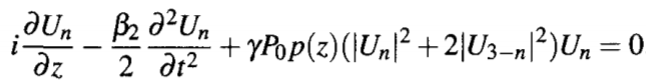where n = 1 or 2. Clearly, the last term is due to XPM. If we ignore the effects of GVD for the moment, this term shows that, over a short distance Δz, the phase of each pulse is shifted nonlinearly by the other pulse by the amountAs this phase shift depends on pulse shape, it varies across the pulse and produces a frequency chirpThis frequency chirp is known as the XPM-induced chirp.

Similar to the case of ASE-induced frequency shift discussed in the "Role of Dispersive and Nonlinear Effects" tutorial, an XPM-induced shift in the carrier frequency of the pulse translates into a shift in the pulse position through changes in the group velocity of the pulse. If all pulses were to shift in time by the same amount, this effect would be harmless. However, the time shift depends on the pattern of bits surrounding each pulse that varies from bit to bit depending on the data transmitted. As a result, pulses shift in their respective time slots by different amounts, a feature referred to as XPM-induced timing jitter. As will be seen later, XPM also introduces some amplitude fluctuations.

A more quantitative estimate of the XPM effects makes use of the moment or the variational method. In this case, we must include the expected frequency and temporal shifts and assume that it has the following solution: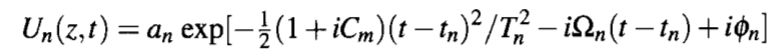where tn represents the position and Ωn represents the frequency shift for the nth pulse. In the moment method, these two quantities are calculated usingwhere E0 is the input energy of each pulse.

Noting that each pulse in the Un(z, t) equation is quantified through six parameters, such an approach leads to 12 first-order differential equations. The two phase equations can be ignored if we neglect interactions that are phase-dependent. The amplitude equations are not needed since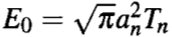which related an to Tn. Furthermore, only the frequency difference ΔΩ = Ω1 - Ω2 and the pulse separation Δt = t1 - t2 are relevant for describing the intrachannel XPM effects. One is thus left with the following set of four equations: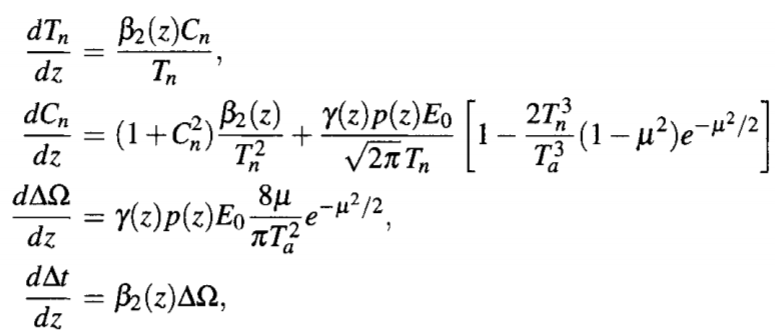where n = 1 or 2, μ = Δt/Ta, and Ta2 = 1/[2(T12 + T22)].

A comparison of the first two equations shows that, although the width equation remains unchanged, overlapping of two neighboring pulses modifies the chirp equation. However, any change in the chirp also affects the pulse width, as the two equations are coupled. Moreover, changes in pulse width would manifest through changes in pulse amplitude sinceremains constant for each pulse. Thus, the amplitude of any 1 bit would depend on whether it is surrounded by 0 or 1 bits. As this pattern varies randomly in an optical bit stream, pulse amplitude would vary from bit to bit. This is the origin of XPM-induced amplitude jitter.

The last two equations show that XPM-induced timing jitter results from the frequency shift ΔΩ. If ΔΩ were to vanish, the pulse separation would remain fixed at its initial value, and no jitter would occur. At a bit rate B, two neighboring pulses are initially spaced by Δt = Tb ≡ 1/B. When both pulses have the same initial width T0, clearly Ta = T0. The third equation above shows that the amount of frequency shift depends on the ratio x = T0/Tb as F(x) = x-3 exp[-1/(2x2)]. The following figure shows how this function varies with x.It peaks near x = 1, indicating that frequency shift ΔΩ is largest when pulse widths are comparable to their temporal spacing. For pulses much smaller than the bit slot, x ≪ 1, and ΔΩ nearly vanishes. This is expected on physical grounds because the tails of such narrow pulses do not overlap significantly, and pulses cannot interact through XPM. What is surprising is that ΔΩ is also relatively small when pulses are much wider than their spacing so that x ≫ 1. Intuitively, one would guess this to be the worse situation since pulses almost completely overlap under such conditions. The reason is related to the fact that the XPM-induced frequency chirp depends on the slope of the pulse power. This slope is smaller for wider pulses and also changes sign, resulting in an averaging effect. The main conclusion is that XPM-induced timing jitter can be reduced by stretching optical pulses over multiple bit slots. This is precisely what is done in pseudo-linear lightwave systems.

The frequency shift ΔΩ and pulse spacing Δt change with z as two pulses propagate inside the fiber link. Such changes are studied by solving the four NLS equations above numerically for a given dispersion map. Figure (a) below shows ΔΩ/2π along a 100-km link formed by using two 50-km fiber sections with D = ±10 ps/(km-nm). The nonlinear parameter γ = 2 W-1/km for both fibers. Losses are assumed to be compensated through distributed amplification so that p(z) = 1. Two Gaussian pulses have 5-ps width (FWHM) at the input and are separated by 25 ps. The solid curve shows the case in which pulses are launched at the input end of the section with anomalous GVD. The almost coinciding dashed curve corresponds to the case in which the dispersion map is made symmetric by launching pulses at the midpoint of this section. Stars and circles show the results obtained in these two cases by solving the NLS equation directly. Most of the frequency shift occurs near the two ends of the map period where pulses are relatively short and overlap only partially.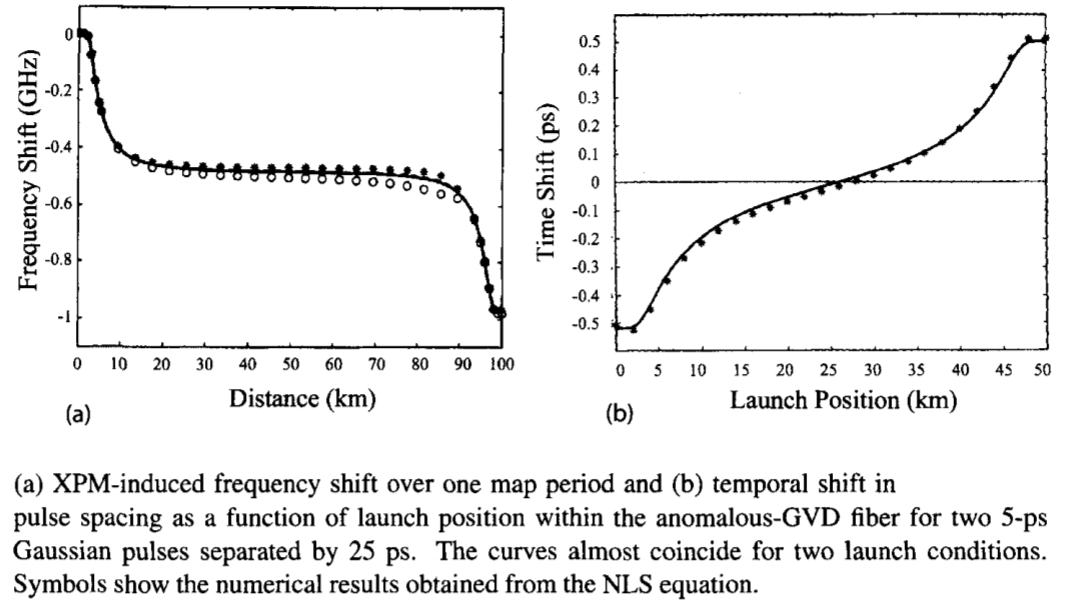Even though the frequency shift is nearly the same for the symmetric and asymmetric maps, the shift in pulse spacing Δt from its initial 25-ps value depends considerably on the exact location where input pulses are launched. This is evident from figure (b) above that shows this shift as a function of the launch position within the anomalous-GVD fiber. Timing shift can be positive or negative depending on whether the first fiber section exhibits anomalous or normal dispersion. The reason is related to the fact that two pulses attract each other in the case of anomalous GVD but they repel each other for normal GVD. As the frequency shift in figure (a) above grows monotonically, it is the second section that produces the most shift. The most important feature of this figure is that pulse position does not shift at all for a symmetric dispersion map. In this case, timing shifts produced in the two sections completely cancel each other. This cancellation can occur only when distributed amplification is used, and we can set p(z) ≈ 1. In the case of lumped amplification, large power variations make the XPM effects much stronger in the first section of a periodic dispersion map.

When lumped amplification is used, the dispersion map is not symmetric. However, it is possible to cancel the XPM-induced time shift by suitably chirping input pulses before launching them into the fiber link. Experimentally, a fiber of suitable length is used to chirp the pulse. This technique is equivalent to the precompensation technique discussed in the dispersion compensating fiber tutorial. The following figure shows the frequency and temporal shifts acquired after one map period as a function of fiber length used for precompensation. The 100-km-long map consists of a 75-km section with β2 = -5 ps2/km, followed with a 25-km DCF section with β2 = 20 ps2/km. The same DCF is used for precompensation. Even though there is always a net frequency shift after one map period, the temporal shift vanishes when the length of precompensation fiber is about 3 km.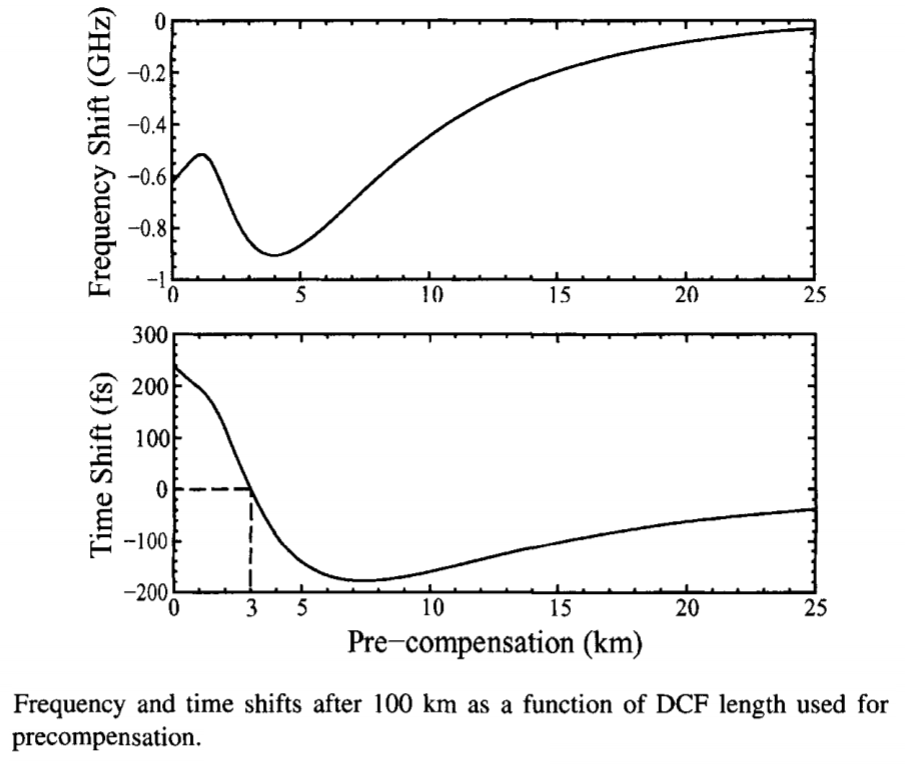#### 3. Intrachannel Four-Wave Mixing (FWM)

The impact of intrachannel FWM is quite different from intrachannel XPM because this nonlinear process can transfer energy from one pulse to neighboring pulses. In particular, it can create new pulses in bit slots that represent 0's and contain no pulse initially. Such FWM-generated pulses (called ghost or shadow pulses) are undesirable for any lightwave system because they lead to additional errors if their amplitude becomes substantial. Ghost pulses were observed as early as 1992 when a pair of ultrashort pulses, each stretched to 90 ps, was propagated through an optical fiber. However, this phenomenon attracted attention only after 1999 when it was found to impact the performance of lightwave systems employing strong dispersion management.

As an example of the system degradation caused by intrachannel FWM, the following figure shows the results of numerical simulations for a 40-Gb/s system at the end of a 80-km-long standard fiber with D = 17 ps/(km-nm). The 5-ps Gaussian input pulses were first chirped by propagating them through a precompensation fiber with DL = -527 ps/nm. Other parameters are identical to those used for the last figure. Because of the rapid broadening of input pulses, timing jitter is reduced considerably. However, ghost pulses appear in all 0 slots, and they degrade the eye diagram considerably. Amplitude fluctuations seen in this figure also result from intrachannel FWM.Compared with the case of XPM, analytic treatment of intrachannel FWM is more involved. A perturbative approach has been used with considerable success to describe the impact of intrachannel nonlinearities, but its accuracy decreases rapidly for large timing jitter. Its main advantages are: one does not need to assume a specific pulse shape and it can be extended easily even to the case of a pseudorandom bit stream. The main idea is to assume that the solution of the NLS equation can be written in the form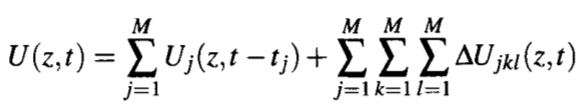where M is the number of bits, Uj represents the amplitude of the jth bit located at t = tj initially, and ΔUjkl is the perturbation created by the nonlinear term. The first term represents the zeroth-order solution obtained by neglecting the nonlinear term in the NLS equation (γ = 0). This solution can be obtained in an analytic form. The second term represents the contribution of all nonlinear effects. It can also be obtained in a closed form by employing first-order perturbation theory.

In the case of Gaussian input pulses of width T0, the perturbation produced by the nonlinear term is given by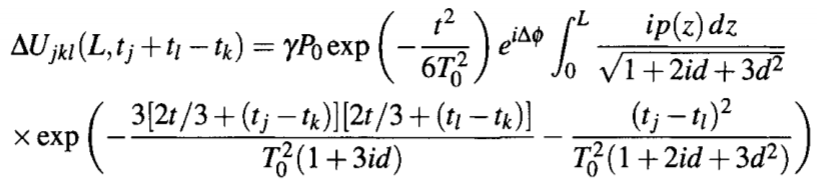where Δφ = φk + φl  - φj is related to the phases of individual pulses and the parameter d(z) is defined as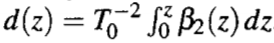All intrachannel nonlinear effects produced by SPM, XPM, and FWM are included in this perturbative solution.  The number of terms that must be included in the triple sum in the U(z, t) equation scales as M3 for a bit stream with M bits. The integral in the ΔUjkl equation can be performed analytically in some limiting cases. For example, if we consider a constant-dispersion fiber, set p(z) = 1 assuming ideal distributed amplification, and consider a fiber length L much longer than the dispersion length LD = T02/|β2|, we obtainwhere rjkl = (tj - tk)(tl - tk)/T02 and E1(x) represents the exponential integral function.

Further insight can be gained by considering the simplest case of two pulses located at t1 = Tb and t2 = 2Tb. In this case, j, k, and l take values 1 or 2, and 8 terms are contained in the triple sum. The SPM effects are governed by the combinations 111 and 222. The effects of intrachannel XPM are governed by the four combinations 112, 122, 211, and 221. The remaining two combinations, 121 and 212, produce intrachannel FWM and perturbations that are located not at the original position of the input pulses but at locations 0 and 3Tb. If these two time slots contain pulses (and represent 1 bits), this perturbation beats with them and manifests as amplitude jitter. In contrast, if they represent 0 bits, a ghost pulse appears in these time slots.

Ghost pulses impact the detection process considerably depending on their power level. The peak power of the ghost pulse located at t = 0 is found from the last equation, after setting j = l = 1, and k = 2, to be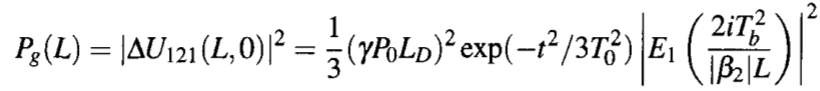where we used t1 = Tb and t2 = 2Tb for the location of two pulses whose overlapping generated the ghost pulse through intrachannel FWM. The figure below shows (a) how the peak power grows with the link length L for a 40-Gb/s signal (Tb = 25 ps) and (b) how it decreases at L = 20 km with the duration Tb of the bit slot, when a bit stream is launched with 10-mW average power into a link made of standard fibers. The dotted curve is obtained when E1(x) is replaced with its asymptotic approximation in which |E1(x)| ~ ln(|1/x|). Such a logarithmic growth of Pg with L holds only for links with constant dispersion. The power transferred to ghost pulses can be reduced by increasing Tb. Indeed, the equation above predicts that Pg varies with Tb as Tb-4 when we use an asymptotic approximation in which |E1(x)| ~ 1/x for large value of x. As seen in the figure below, the predictions of this equation are in good agreement with the numerical simulations based on the NLS equation.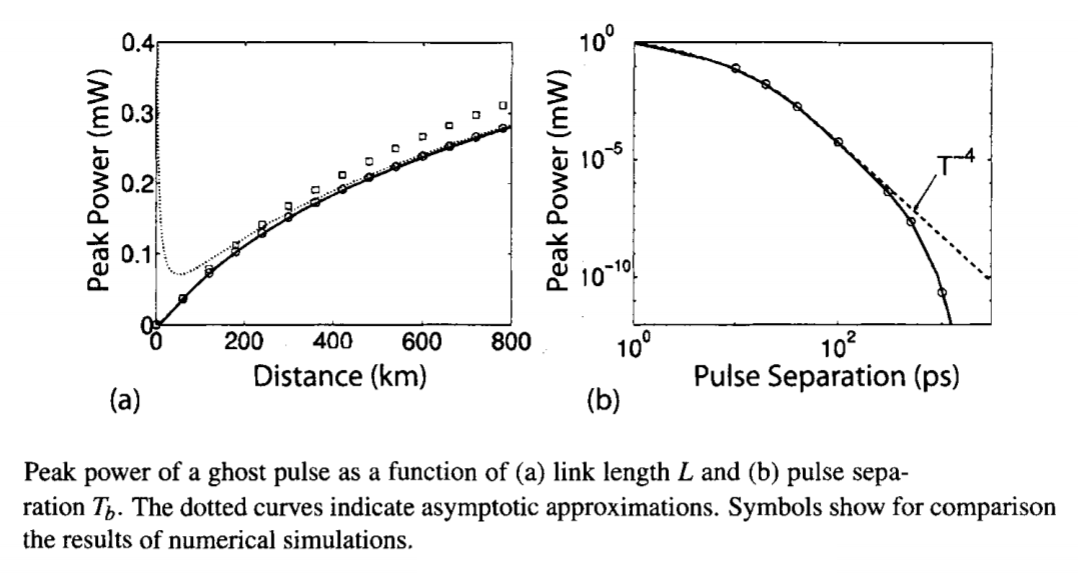The preceding results change considerably when a periodic dispersion map is employed. Even though peak power or energy of each ghost pulse grows in a logarithmic fashion during a single map period, it can still build up rapidly along the link because of a resonance related to the periodic nature of loss and dispersion variations. Physically speaking, the amplitudes of ghost pulse generated in each map period add up in phase because of this resonance. As a result, the total peak power at the end of a link length L grows as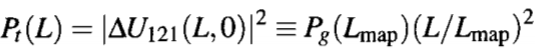where Lmap is the map period. Such quadratic growth of the ghost-pulse energy becomes of considerable concern for long-haul systems.

Intrachannel FWM also leads to amplitude fluctuations. Physically speaking, whenever the perturbation ΔUjkl falls within the bit slots occupied by 1 bits, it beats with the amplitude of that bit. This beating modifies the amplitude of each 1 bit by an amount that depends not only on the pseudo-random bit pattern but also on the relative phases of neighboring pulses. In the case of a periodic dispersion map, energy fluctuations grow only linearly with length of the fiber link. Moreover, they can be reduced considerably by adopting a distributed amplification scheme such that the average power does not vary much along the link.